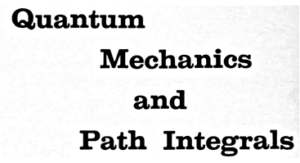# 問題 6-23 の解答例Problem 6-23
Show that the same result is obtained for $$\newcommand{\mb}{\mathbf{#1}} d\sigma/d\Omega$$ even if the wave functions $$\phi_n$$ are normalized to give a unit probability for some arbitrary volume $$V$$.

( 解答 )　§ 4.3 の式 (4-72) と式 (4-73) における説明のように, 体積 $$V$$ で規格化された平面波 $$\displaystyle{\frac{1}{\sqrt{V}}\exp(i\mb{k}\cdot\mb{r})}$$ を使う場合, $$\mb{k}$$-空間に於ける領域 $$d^{3}\mb{k}$$ 中の状態数は $$\displaystyle{V\frac{d^{3}\mb{k}}{(2\pi)^{3}}}$$ であるので， $$\sum_{\mb{p}}$$ は $$\displaystyle{V\int \frac{d^{3}\mb{p}}{(2\pi\hbar)^{3}}}$$ で置き換えなければならないのであった． よって, 式 (6-90) は次のように書き換えるべきである：

\begin{equation}
\tag{1}
\end{equation}

\begin{equation}
\rho(E)=\frac{V}{dE}\frac{p^{2}\,dp\,d\Omega}{(2\pi\hbar)^{3}}=\frac{V m}{p\,dp}\frac{p^{2}\,dp\,d\Omega}{(2\pi\hbar)^{3}}
=\frac{V}{(2\pi\hbar)^{3}} m p\,d\Omega
\tag{2}
\end{equation}

また上述より, 式 (6-88) の「行列要素」 $$V_{mn}$$ も次のように書くべきである：
\begin{align}
V_{mn}&=\int d^{3}\mb{r}\,\frac{1}{\sqrt{V}}e^{-i\mb{p}_2\cdot\mb{r}/\hbar}\,V(\mb{r})\,
\frac{1}{\sqrt{V}}e^{i\mb{p}_1\cdot\mb{r}/\hbar}
=\frac{1}{V}\int d^{3}\mb{r}\,e^{-i\mb{p}_2\cdot\mb{r}/\hbar}\,V(\mb{r})\,e^{i\mb{p}_1\cdot\mb{r}/\hbar}\notag\\
&=\frac{1}{V}\,v(\mb{q})
\tag{3}
\end{align}

さらに, 単位体積当たり 1 個の粒子を見出す相対確率は $$\displaystyle{\frac{1}{V}}$$ となる． よって式 (6-92) は次のように修正される：
\begin{equation}
\frac{d P}{d t}=\frac{1}{V} u_1\,d\sigma=\frac{1}{V}\frac{p}{m}d\sigma
\tag{4}
\end{equation}

\begin{equation}
\frac{d\sigma}{d\Omega} = \frac{V m}{p}\cdot\frac{2\pi}{\hbar}\cdot\frac{|v(\mb{q})|^{2}}{V}
\cdot\frac{m p}{(2\pi\hbar)^{3}}
\tag{5}
\end{equation}

\begin{equation}
\frac{d\sigma}{d\Omega}=\left(\frac{m}{2\pi\hbar^{2}}\right)^{2}|v(\mb{q})|^{2}
\tag{6}
\end{equation}

これには体積因子 $$V$$ が含まれておらず, 式 (6-93) と同じ結果となっている．

Show that the same result is obtained for $$d\sigma/d\Omega$$ if the wave functions $$\phi(\mb{x})$$ have the specific normalization of unity for a box of unit volume, e.g., $$\phi_n(\mb{x})= e^{i(\mb{p}_{a}/\hbar)\cdot\mb{x}}$$.
これは校訂版では, 式 (6-87) 後よりこの問題 6-23 まで, 式や文章の表現が大分書き換えてあるからである．
しかし, ファインマンがこの問題を提起したのは「結果式である式 (6-93) に体積 $$V$$ が出現しないのは尤もなこと」であることを示しておきたかったからではなかろうか？． それは,「散乱断面積の式 (6-93) を求めるとき, 入射粒子を平面波と見做し, その際, その平面波には周期的境界条件を課して大きさ $$V$$ の箱の中で規格化されているとした． しかしこの体積 $$V$$ はあくまで計算上の便宜のために人工的に導入したものだから」である． よって, ここで示す解答例は原書の文章に沿ったものとするので気を付けて欲しい．

Some Remarks on Mathematical Rigor.　The reader may have one or two reactions on seeing how the volumes $$V$$ cancel at the end of this calculation. One might be: How nicely it cancels out as it should, for the walls have no effect. The other might be: Why do it in this complicated and “dirty” non rigorous manner, putting in walls which make no difference, etc., when all this can be done much more elegantly and rigorously mathematically without the need of walls, etc? It depends whether you are physically minded or mathematically minded. There are many misunderstandings between mathematicians and physicists on the place of mathematical rigor in physics, so perhaps a word as to the value of each method ( the box or mathematical rigor ) may be in order.
There is, of course, the more trivial point: Which is most familiar — which takes the least new knowledge? Most physicists have seen this argument about how to count the states in a box before.
Another point is that the mathematically rigorous solution may not be physically rigorous. That is, the box may in fact exist. It may not be a rectangular box, but it is not often that experiments are done under the stars. Rather they are done in a room. Although it is physically reasonable that the walls have no effect, it is true that the original problem is set up as an idealization. It is no more satisfactory idealization to move the walls to infinity than to replace them by perfect mirrors far away. The mathematical rigor is wasted in the first idealization, since the walls are not at infinity.
The wall approach is just as rigorous, or rather just as nonrigoryous. It has several advantages, For example, in finding that the volume cancels out we do learn that at least one aspect of the idealized walls, namely how far away they are, is unimportant. This discovery makes us more intuitively convinced that the actual dispositon of the real environment may be unimportant. Finally, the formula derived is very useful when in fact we do have a finite sample. For example, in Chap. 8 we shall use it to count sound-wave modes in a large, rectangular block of material.
On the other hand, the advantage of the mathematically clean argument is the avoidance of much unnecessary detail that cancels out. Although, using the wall approach, one may learn something about how the walls have no effect, one may be firmly convinced that this is true anyway and not wish to depend into details to see it again.
The normalization problem is a special example, but it illustrates the point. The physicist can not understand the mathematician’s care in solving an idealized physical problem. The physicist knows the real problem is much more complicated. It has already been simplified by intuition, which discards the unimportant and often approximates the remainder.

シェアする
イッシーをフォローするファインマンさんの肩に乗って